##The dual conic of the incircle

This is a particular case from the duality mentioned in TriangleProjectivitiesPlay.html and explained in TriangleConics.html . The conic c under consideration is the dual circumscribed to triangle ABC, when the inscribed conic coincides with the incircle. Several facts are related to this conic (could be called the Soddy conic of the triangle, see  below), some of them listed below.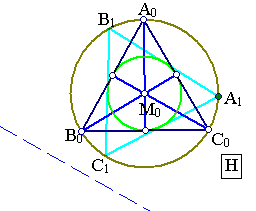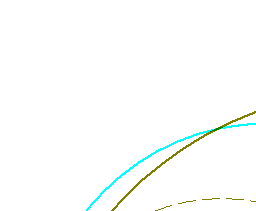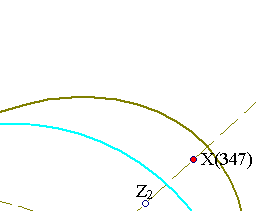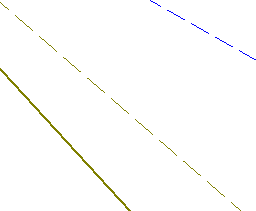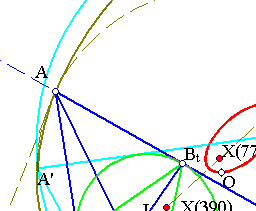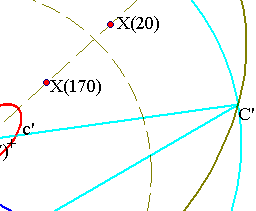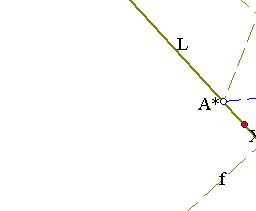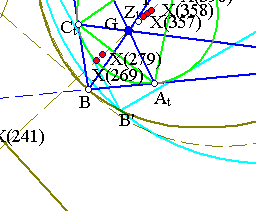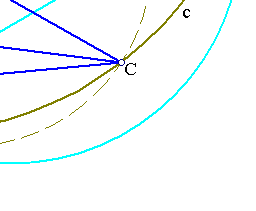Conic c can be obtained as the image of the circumcircle of the equilateral A0B0C0 through the projectivity H mapping the vertices A0, B0, C0 to A, B, C correspondingly and the center M0 of the equilateral to the Gergonne point G. These requirements completely determine H, which then maps the incircle of the equilateral to the incircle of ABC. The point named x in the cited reference is here the Gergonne point G and the corresponding trilinear polar is denoted here by L. L coincides with the Gergonne line of the triangle (naming it after Oldknow).
 The conic c can be elliptic, parabolic or hyperbolic, depending on the shape of the triangle. Its focal points Z1, Z2 are on line (f) passing through G and I. Z1, Z2 coincide with the inner/outer Soddy points of the triangle.
 Line f is orthogonal to L (called the Soddy line of the triangle). f coincides with the Brocard axis of the contact triangle AtBtCt with vertices at the contact points of incircle with the sides of ABC.
 Line L coincides with the Lemoine axis of triangle AtBtCt and carries, among others, the triangle-center X(241), which coincides with X(237) relative to AtBtCt.
 Line f carries several triangle centers. For example: the De Longchamps point X(20), X(77), X(170), X(269), X(279), X(347), X(357), X(358), X(390).
 Consider another equilateral A1B1C1 resulting by rotating A0B0C0 about its center. Then apply H to get triangle A'B'C'. This triangle shares with ABC several geometric characteristics such as:
[6.1] Same incircle, and conjugate conic, same Gergonne point G, incenter I, lines f and L.
[6.2] Triangle centers of A'B'C' lying on f either remain the same or move on line f. In particular the Soddy points of all triangles A'B'C' are the same.
[6.3] Triangle centers of A'B'C' lying on L either remain fixed or move on line L.
 The circumcenters of A'B'C' describe another conic c', not belonging to the family generated by the incircle and its conjugate conic c, sharing though with c one axis.

CircumcircleConjugate.html
CrossRatio.html
CrossRatio0.html
CrossRatioLines.html
GoodParametrization.html
Harmonic.html
Harmonic_Bundle.html
HomographicRelation.html
HomographicRelationExample.html
Pascal.html
PascalOnTriangles.html
ProjectivityFixingVertices.html
ProjectiveRotations.html
Steiner_Ellipse.html
TriangleCircumconics.html
TriangleCircumconics2.html
TriangleConics.html
TriangleProjectivitiesPlay.html
TrilinearPolar.html

### References

Oldknow, A. The Euler-Gergonne-Soddy triangle of a triangle The Am. Math. Monthly vol. 103(1996) pp. 319-329
 Produced with EucliDraw© http://users.math.uoc.gr/~pamfilos/